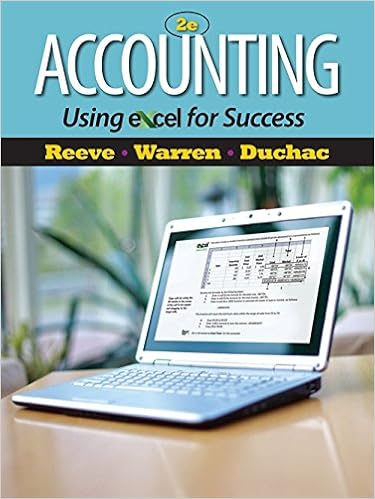# A credit balance in the labor efficiency variance

• Test Prep
• 13
• 90% (10) 9 out of 10 people found this document helpful

This preview shows page 3 - 5 out of 13 pages.

##### We have textbook solutions for you!
The document you are viewing contains questions related to this textbook.The document you are viewing contains questions related to this textbook.
Chapter 19 / Exercise 19-7
Accounting Using Excel for Success
Reeve/WarrenExpert Verified
26. A credit balance in the labor efficiency variance indicates that: A. standard hours exceed actual hours B. actual hours exceed standard hours C. standard rate and standard hours exceed actual rate and actual hours D. actual rate and actual hours exceed standard rate and standard hours
27. If the actual labor rate exceeds the standard labor rate and the actual labor hours exceed the number of hours allowed, the labor rate variance and labor efficiency variance will be
D.
28. In the analysis of standard cost variances, the item which receives the most diverse treatment in accounting is
29. When expenses estimated for the capacity attained differ from the actual expenses incurred, the resulting balance is termed the
##### We have textbook solutions for you!
The document you are viewing contains questions related to this textbook.The document you are viewing contains questions related to this textbook.
Chapter 19 / Exercise 19-7
Accounting Using Excel for Success
Reeve/WarrenExpert Verified
MSQ-04 Page 430. The total overhead variance is A. The difference between actual overhead costs and budgeted overhead. B. Based on actual hours worked for the units produced. C. The difference between actual overhead costs and applied overhead. D. The difference between budgeted overhead and applied overhead.
31. Management scrutinizes variances because
32. If a company uses a predetermined rate for absorption of manufacturing overhead, the volume variance is
33. The production volume variance occurs when using the
•••